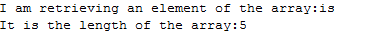# 1D Array in JAVA

## 1D array in JAVA

In this tutorial you will learn 1) What is array in JAVA? 2) Why we use arrays? 3) How we use arrays in JAVA coding? What is an Array? Array is a data structure used in programming languages to store similar type of items. Arrays are the simplest data structure. The contents of array can be quickly accessed and can easily be deleted. Arrays are used to put in independent variables of the same type in them. Array is just like a one directional block having equal sized partitions and each block we have a value stored. Each value in the array is called the element. Compared to C programming the arrays in the JAVA programming are not static rather they have a dynamic nature. Why we use arrays? The arrays help us to store same type of objects in a same place. Each array can be accessed through an index which let us work in a more easy fashion. The index does not contain any value rather it only points towards the location. It provides the faster access in a more organized fashion. Lots of values are stored in a single variable so that the programmer does not need to remember the names of multiple variables. Arrays have a known length, once the size is set, it can not be changed How we use arrays in JAVA coding? Step 1: There are two ways, by using first method we can first declare an array and then in the next part we initialize it.
`//first methodInt[] mynums; //here we have declared an array named ‘mynums’mynums=new int; //here we have given the size of the array name ’mynums’`
By using second method we declare the array first and initialize the array in the same line.
`//second methodInt[] mynums=new int[];`
Step 2: So the first part is about making an array, below the example will let us know how we work to insert values into the array.
`mynums = 2; //zeroth location of array ‘mynums’ will have value 2.mynums = 3; mynums = 5; mynums = 7; mynums=2;mynums=23;`
We can also simply give in values to the array and array will determine its size itself. For Example
`int[] mynums = { 10, 100, 1000 }; //here array named ‘mynums’ will have value 10 at zeroth position,//100 at the first position w.r.t index which is always at the start of the array.                                                                                                                      `
We can easily retrieve data from array , you can clearly see the example below
`System.out.print(mynums);System.out.print(mynums);//so on, depending on the number of entries in the array//for loop can also be used`
Code Example
`package tutorial;import java.util.*;public class myclass { 	public static void main(String[] args) {		String[] test = { "This", "is", "a","Java","tutorial"}; //input		System.out.print("I am retrieving an element of the array:"); //just for printing	System.out.print(test); // printing out the 1st element(not zeroth)	   System.out.println(); 		System.out.print("It is the length of the array:");		System.out.print(test.length); // a function can be used to get //length of the array 	} }`
In this code I have demonstrated how we have worked with the elements of the array, first inserted them and then retrieved them. Screen Shot